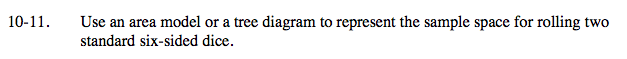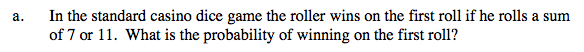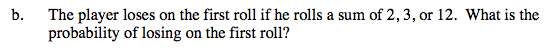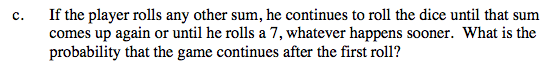### Home > A2C > Chapter Ch10 > Lesson 10.1.1 > Problem10-11

10-11.
1. Use an area model or a tree diagram to represent the sample space for rolling two standard six-sided dice. Homework Help ✎

1. In the standard casino dice game the roller wins on the first roll if he rolls a sum of 7 or 11. What is the probability of winning on the first roll?

2. The player loses on the first roll if he rolls a sum of 2, 3, or 12. What is the probability of losing on the first roll?

3. If the player rolls any other sum, he continues to roll the dice until that sum comes up again or until he rolls a 7, whatever happens sooner. What is the probability that the game continues after the first roll?There are 6 ways to get a sum of 7, and 2 ways to get 11.

$P\text{(7\,or\,11)=\frac{8}{36}=\frac{2}{9}}$$\frac{1}{9}$$\frac{2}{3}$